## MTSO March News - Spring Ahead!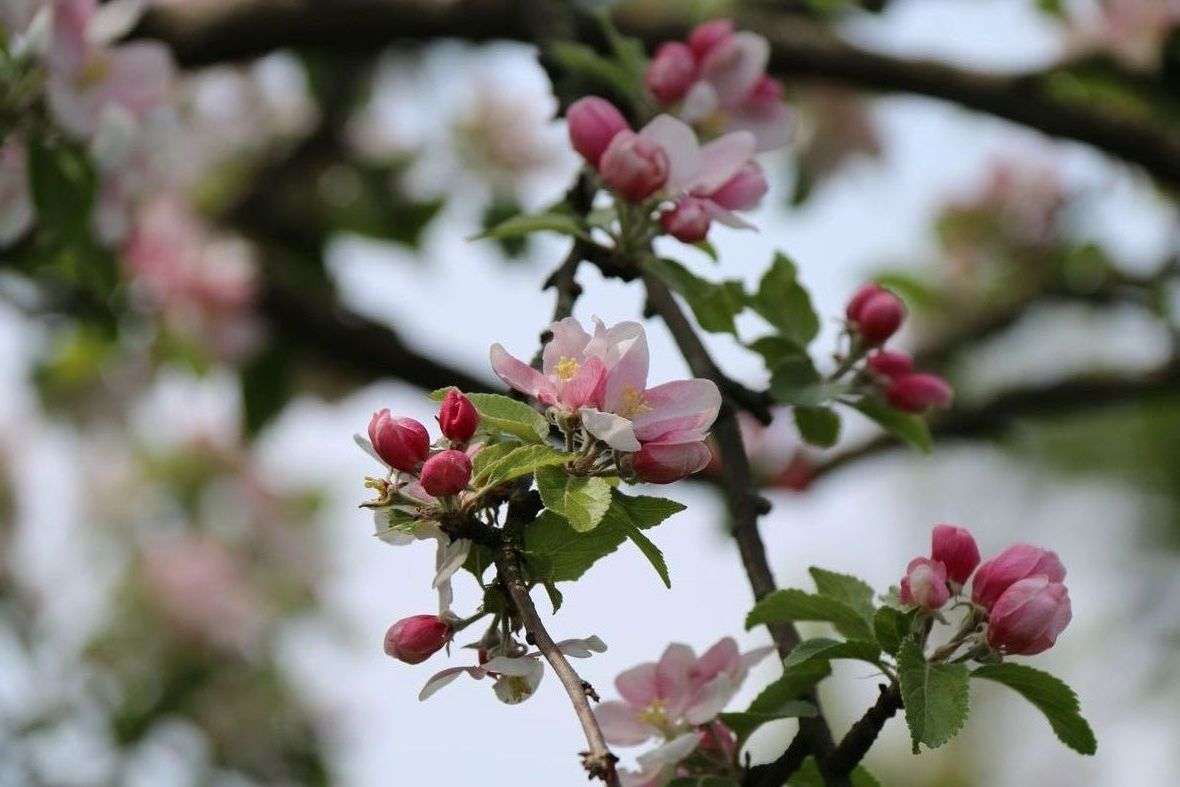March finally arrives, with the anticipation of spring and new beginnings. We are thinking of the Earth as our body. Protecting our earth as we protect our own Bodies, and we have just the products you need! We're featuring the Best Recycling Program Contest, Massage Table specials for students, Aromatherapy for Immune support. Plus: Pillager's Potion, a new size is available. Also, we're featuring Oakworks Tilt top Tables and 3-piece Body Cushions. Scroll down for more....

 table div table+table+table+table div table{width:100%;padding:0}table div table+table+table+table div table img{width:96.23%;padding:0;float:none}table div table+table+table+table div table td{width:100%;padding:0 1.88% 18px}/* styles */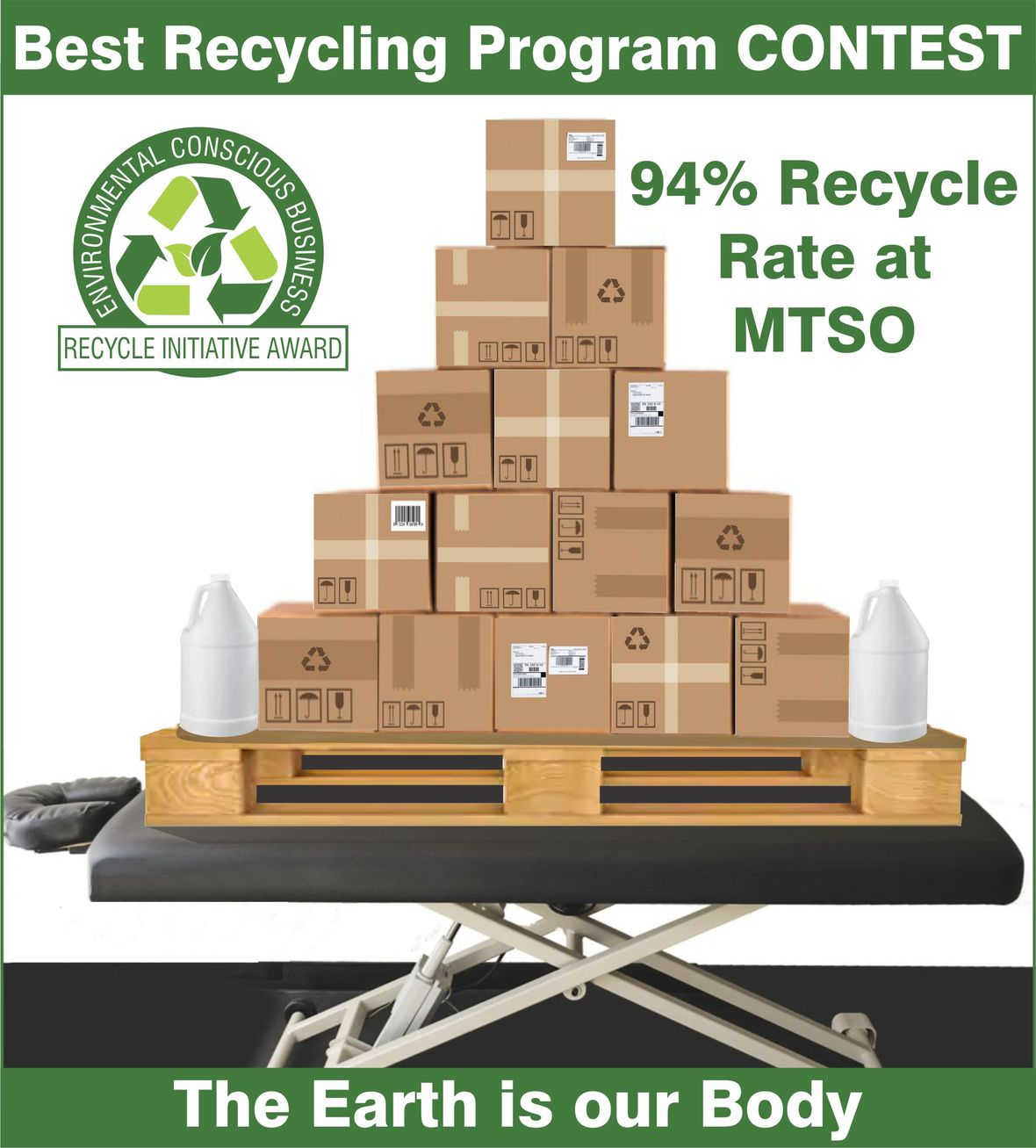# Best Recycling Program CONTEST

94% Recycle Rate at MTSO. We are encouraging Therapists to take care of the Earth by Recycling, Reusing, Re-purposing. Now is the time to develop your own recycling program. Show us your results and have a chance to WIN \$250 worth of Sheets.
Enter the contest by submitting photos and a description of your Recycle Program to: shop@mtso.ab.ca

 table div table+table+table+table+table+table div table{width:100%;padding:0}table div table+table+table+table+table+table div table img{width:96.23%;padding:0;float:none}table div table+table+table+table+table+table div table td{width:100%;padding:0 1.88% 18px}/* styles */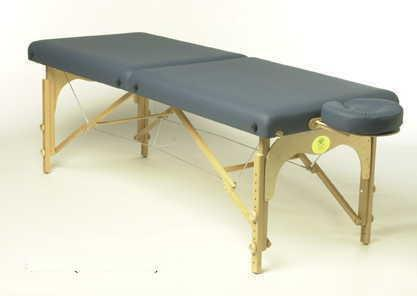Prairie Portable Massage Table

# Massage Student Special

Prairie Portable Massage Table special for massage students. Show your student ID to receive a price of \$485, down from \$510! Phone the store for details.

 table div table+table+table+table+table+table+table+table+table div table{width:100%;padding:0}table div table+table+table+table+table+table+table+table+table div table img{width:96.23%;padding:0;float:none}table div table+table+table+table+table+table+table+table+table div table td{width:100%;padding:0 1.88% 18px}/* styles */## Aromatherapy for Immune Support

 /* styles */ Did you know that Aromatherapy can support your immune system during this season of cold, flu & virus worries? Last month this newsletter we mentioned Eucalyptus for respiratory & congestion relief. This month we want to mention some of the aromatherapy blends that can be added to an unscented oil, gel or lotion, which support immune function. These include CF (cold & flu), MJ (muscle & joint), RF (refresh blend), and Tranquil Synergy.
 /* styles */ Click on image to purchase
 table div table+table+table+table+table+table+table+table+table+table+table+table+table+table+table div table{width:100%;padding:0}table div table+table+table+table+table+table+table+table+table+table+table+table+table+table+table div table img{width:96.23%;padding:0;float:none}table div table+table+table+table+table+table+table+table+table+table+table+table+table+table+table div table td{width:100%;padding:0 1.88% 18px}/* styles */# Pillager's Potion - Now Available in a 30ml size

Pillager's Potion for immune system support during "cold & flu season". It's an easy & pleasant blend to diffuse, & also very supportive applied during massage (1-2 drops at a time in about 1tsp of oil or lotion) to lymphatic drainage areas, & aching muscles. It's warming & decongestive to all body systems.

This is a superb blend to diffuse into your reception area to reduce airborne microbes. It's been such a popular item that we are now carrying a larger 30ml bottle, as well as the original 10ml size.

 /* styles */ Click on image to purchase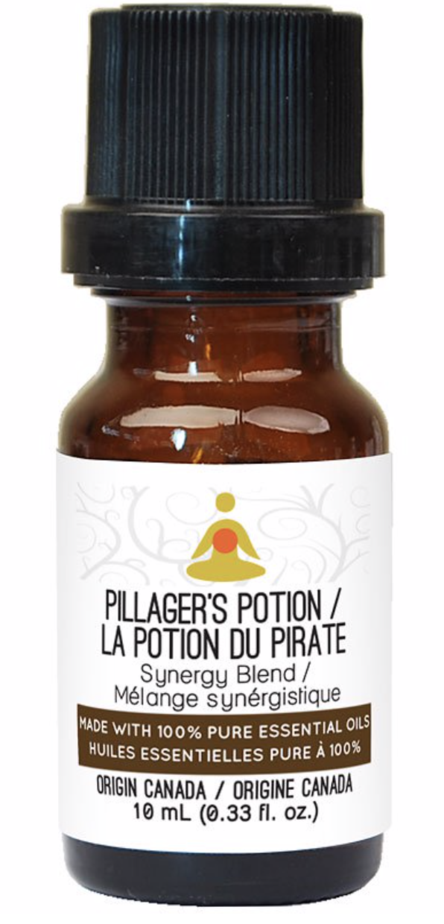Pillager's Potion Essential Oil Blend 10ML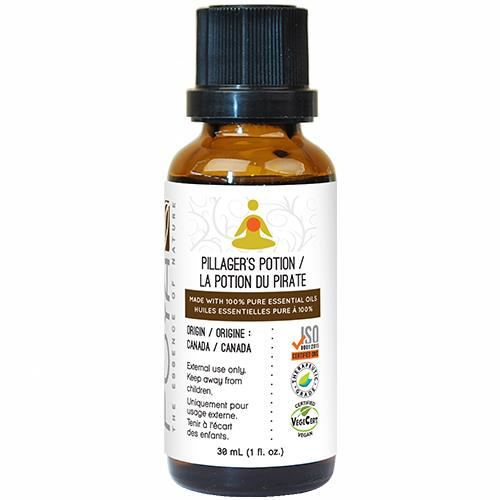Pillager's Potion Essential Oil Blend 30ML
 table div table+table+table+table+table+table+table+table+table+table+table+table+table+table+table+table+table+table+table div table{width:100%;padding:0}table div table+table+table+table+table+table+table+table+table+table+table+table+table+table+table+table+table+table+table div table img{width:96.23%;padding:0;float:none}table div table+table+table+table+table+table+table+table+table+table+table+table+table+table+table+table+table+table+table div table td{width:100%;padding:0 1.88% 18px}/* styles */# Oakworks Tilt Top Table

Customers love this versatile table for therapeutic & spa applications. This table is the perfect choice when you are looking for great value and excellent functionality. The available tops are the same as found on our most expensive tables. Featuring our largest height range, these tables can go down lower than any others in our line.

 table div table+table+table+table+table+table+table+table+table+table+table+table+table+table+table+table+table+table+table+table+table+table div table{width:100%;padding:0}table div table+table+table+table+table+table+table+table+table+table+table+table+table+table+table+table+table+table+table+table+table+table div table img{width:96.23%;padding:0;float:none}table div table+table+table+table+table+table+table+table+table+table+table+table+table+table+table+table+table+table+table+table+table+table div table td{width:100%;padding:0 1.88% 18px}/* styles */table.module-22{width:75.47%;padding:0}table div table+table+table+table+table+table+table+table+table+table+table+table+table+table+table+table+table+table+table+table+table+table+table div table{width:75.47%;float:none;margin-left:auto;margin-right:auto;padding:0}table div table+table+table+table+table+table+table+table+table+table+table+table+table+table+table+table+table+table+table+table+table+table+table div table a{border:0 none;text-decoration:none}table div table+table+table+table+table+table+table+table+table+table+table+table+table+table+table+table+table+table+table+table+table+table+table div table img{width:100%!important;border:0 none;text-decoration:none}table div table+table+table+table+table+table+table+table+table+table+table+table+table+table+table+table+table+table+table+table+table+table+table div table td{width:100%;padding:0}/* styles */

# 3-Piece Body Cushion

Increase the comfort of your "Mommies-to-be" & post-surgical clients. The bodyCushion yields better, faster results in treating clients than a flat table. Patented, specific-density construction provides optimal support for reducing pressure and tension in your clients' bodies so that your work will be more effective.

 table div table+table+table+table+table+table+table+table+table+table+table+table+table+table+table+table+table+table+table+table+table+table+table+table+table+table div table{width:100%;padding:0}table div table+table+table+table+table+table+table+table+table+table+table+table+table+table+table+table+table+table+table+table+table+table+table+table+table+table div table img{width:96.23%;padding:0;float:none}table div table+table+table+table+table+table+table+table+table+table+table+table+table+table+table+table+table+table+table+table+table+table+table+table+table+table div table td{width:100%;padding:0 1.88% 18px}/* styles */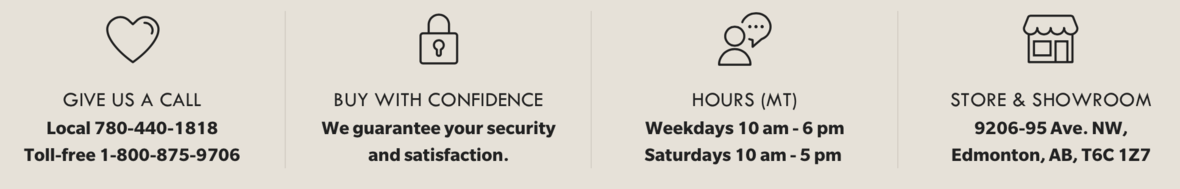## Order online for delivery or pickup

Browse and buy from an extensive collection at our online store, always open, 24/7/365. Edmonton store and showroom: 10:00 - 6:00 Mon-Fri, 10:00-5:00 Sat.

 table.module-28{width:51.32%;padding:0}table div table+table+table+table+table+table+table+table+table+table+table+table+table+table+table+table+table+table+table+table+table+table+table+table+table+table+table+table+table div table{width:51.32%;float:none;margin-left:auto;margin-right:auto;padding:0}table div table+table+table+table+table+table+table+table+table+table+table+table+table+table+table+table+table+table+table+table+table+table+table+table+table+table+table+table+table div table a{border:0 none;text-decoration:none}table div table+table+table+table+table+table+table+table+table+table+table+table+table+table+table+table+table+table+table+table+table+table+table+table+table+table+table+table+table div table img{width:100%!important;border:0 none;text-decoration:none}table div table+table+table+table+table+table+table+table+table+table+table+table+table+table+table+table+table+table+table+table+table+table+table+table+table+table+table+table+table div table td{width:100%;padding:0}/* styles */
 Like   Tweet   Pin   in